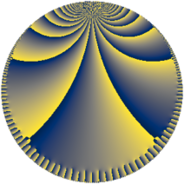# Properties

 Label 252.9.rLevel $252$ Weight $9$ Character orbit 252.r Rep. character $\chi_{252}(131,\cdot)$ Character field $\Q(\zeta_{6})$ Dimension $760$ Sturm bound $432$

# Related objects

## Defining parameters

 Level: $$N$$ $$=$$ $$252 = 2^{2} \cdot 3^{2} \cdot 7$$ Weight: $$k$$ $$=$$ $$9$$ Character orbit: $$[\chi]$$ $$=$$ 252.r (of order $$6$$ and degree $$2$$) Character conductor: $$\operatorname{cond}(\chi)$$ $$=$$ $$252$$ Character field: $$\Q(\zeta_{6})$$ Sturm bound: $$432$$

## Dimensions

The following table gives the dimensions of various subspaces of $$M_{9}(252, [\chi])$$.

Total New Old
Modular forms 776 776 0
Cusp forms 760 760 0
Eisenstein series 16 16 0

## Trace form

 $$760 q - 2 q^{4} - 6 q^{5} - 768 q^{6} - 2 q^{9} + O(q^{10})$$ $$760 q - 2 q^{4} - 6 q^{5} - 768 q^{6} - 2 q^{9} - 6 q^{10} + 83142 q^{12} + 26502 q^{14} - 2 q^{16} - 143902 q^{18} - 157730 q^{21} - 514 q^{22} - 19686 q^{24} - 28437498 q^{25} + 768 q^{26} + 65532 q^{28} - 1897644 q^{29} + 1785905 q^{30} - 6 q^{33} + 768 q^{34} + 4312090 q^{36} - 4 q^{37} - 9654033 q^{38} + 1171872 q^{40} + 9432706 q^{42} - 18134733 q^{44} + 4739994 q^{45} - 514 q^{46} + 19683 q^{48} - 2 q^{49} - 1171881 q^{50} - 393219 q^{52} + 25242027 q^{54} - 17294406 q^{56} + 9885756 q^{57} + 513 q^{58} + 24314273 q^{60} - 1536 q^{62} - 8 q^{64} - 31205016 q^{66} + 26573172 q^{68} + 39366 q^{69} + 12310595 q^{70} - 39352510 q^{72} - 12 q^{73} + 146576199 q^{74} + 196608 q^{76} - 35105478 q^{77} + 57053751 q^{78} + 258235821 q^{80} - 195701762 q^{81} + 762 q^{82} - 310058731 q^{84} + 781246 q^{85} - 596778015 q^{86} + 16777217 q^{88} - 220940640 q^{89} - 326303607 q^{90} + 281307144 q^{92} + 68073270 q^{93} + 224825871 q^{96} + 450938739 q^{98} + O(q^{100})$$

## Decomposition of $$S_{9}^{\mathrm{new}}(252, [\chi])$$ into newform subspaces

The newforms in this space have not yet been added to the LMFDB.